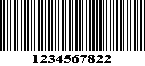## MSI/Plessey Specification

The MSI symbol consists of the following elements: a forward start code, four bars with intervening spaces for each encoded digit, one or two check digits, and a stop code.MSI Code is a numeric, continuous code. Each character consists of four bits, using a straight binary encodation. Each bit is encoded in the following way: if the bit is a 1, the pattern to be used is a wide bar followed by a narrow space. If the bit is to be a 0, the pattern is a narrow bar followed by a wide space. The following table is the bit patterns:

### digit pattern

```     0           0000
1           0001
2           0010
3           0011
4           0100
5           0101
6           0110
7           0111
8           1000
9           1001
```

The start character is a single ``1'' bit. (wide bar/narrow space).
The stop character is a narrow bar/wide space/narrow bar.

### Check digit calculation

The MSI code can include 1 or 2 check digits.
The check digits can be calculated either in Modulo 10 or Modulo 11.

#### Modulo 10 check digit calculation

```Step 1: Designate the least significant (right-most)
digit position as even (E).

5 7 6 3 5 7 9 0 1 2 5   <-- Message digits
O E O E O E O E O E O   <-- Digit positions

Step 2: Form a new number using the odd (O) position
digits in sequence

565915

Step 3: Multiply the new number by 2

565915 * 2 = 1131830

Step 4: Sum all the digits of the result.

1 + 1 + 3 + 1 + 8 + 3 + 0  = 17

Step 5: Sum all the digits in the even positions
and add the result to Step 4.

7 + 3 + 7 + 0 + 2 = 19 + 17 = 36

Step 6: Subtract the result from the next
higher multiple of 10

40 - 36 = 4;

The Modulo 10 check digit is 4.```

#### Modulo 11 check digit calculation

The modulo 11 check digit calculation uses weighting factors which repeat from
right to left in the following sequence: (2,3,4,5,6,7,2,3,4,5,6,7,2,3,4,5,6,7,...)

```Step 1: Starting with the right-most digit, assign a weight
to each digit position.

5 7 6 3 5 7 9 0 1 2 5   <-- Message digits
6 5 4 3 2 7 6 5 4 3 2   <-- weights

Step 2: Calculate and sum the products of each weight
and digit.

(5*6)+(7*5)+(6*4)+(3*3)+(5*2)+(7*7)+
(9*6)+(0*5)+(1*4)+(2*3)+(5*2) = 231

Step 3: Subtract the result from the next higher
multiple of 11

231 - 231 = 0

The Modulo 11 check digit is 0.```